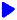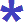Noetic Learning Math Worksheet Creator

Generate Subtraction WorksheetsBack to Worksheet Creator HomeSubtraction up to

in algebra format: solve for

Number of questions:   questions per row: Generate!Subtraction with no regrouping digit minus   digit

in algebra format: solve for

Number of questions:   questions per row: Generate!Subtraction digit  minus   digit   non-negative results only

in algebra format: solve for

Number of questions:  questions per row: Generate!